# Custom Color Ramp Nodes

The default color ramp node in Blender is indeed a very useful node whenever you need to modify range of some color values. However, when creating some versatile node setups, you might be faced with the lack of inputs for the ramp control points. And there is the time to create your custom color ramp node with control inputs.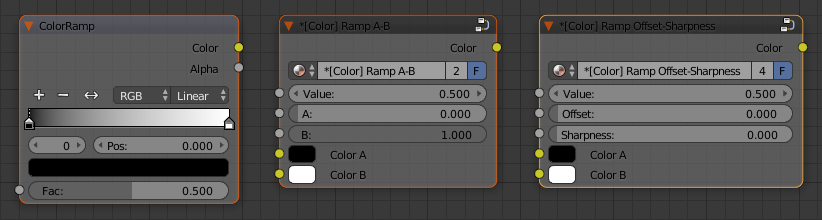Color ramp nodes: Blender's default node and two custom nodes with control inputs.

## Custom Color Ramp Node: A-B

A linear ramp function with control points $a$, $b$ (like on the picture below) can be expressed by a piecewise function

\begin{equation*} f(x) = \begin{cases} 0, & x \leq a \\ \frac{1}{b-a}x - \frac{a}{b-a}, & a<x<b \\ 1, & x \geq b \end{cases} \end{equation*}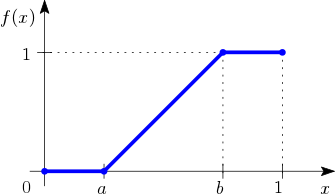Then, the output value can be used to mix two colors by a MixRGB node. Implemented in the node editor it looks like this...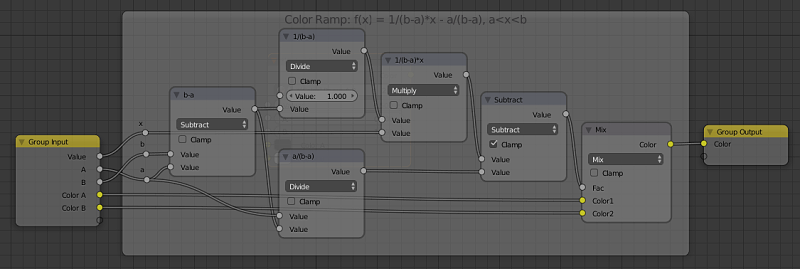Node setup for custom color ramp with control inputs A-B

## Custom Color Ramp Node: Offset-Sharpness

Later, when using the color ramp to adjust procedural textures, I have found that in most cases I don't really need to adjust both control points independently. But rather set value $a$ as an offset of the dark areas and the span $(b-a)$ to define sharpness of the final texture.

More precisely, I've defined the sharpness factor that varies from 0.0 to 1.0 as

\begin{equation*} \xi_{sharpness} = \frac{b-1}{a-1}, \end{equation*}

which means if sharpness is 1.0, then $b=a$ and the color ramp output is 100% sharp (black & white).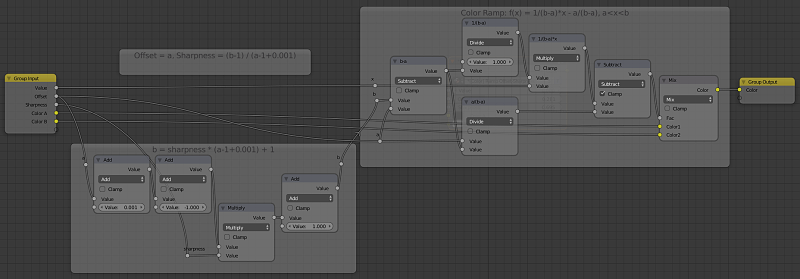Node setup for custom color ramp with control inputs Offset-Sharpness

Demo - Color ramp node applied to the Voronoi procedural texture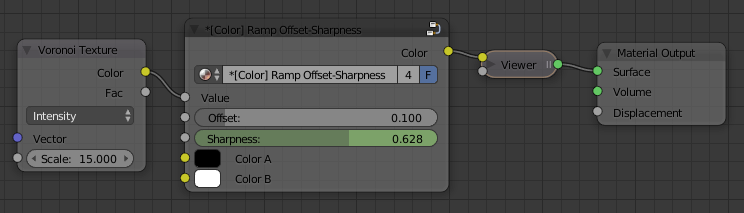Color ramp node applied to the Voronoi procedural texture.

Animated demo (sharpness varies from 0.0 to 1.0 and back).